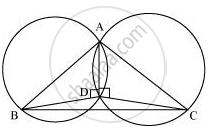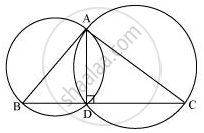# If circles are drawn taking two sides of a triangle as diameters, prove that the point of intersection of these circles lie on the third side. - Mathematics

If circles are drawn taking two sides of a triangle as diameters, prove that the point of intersection of these circles lie on the third side.

#### SolutionConsider a ΔABC.

Two circles are drawn while taking AB and AC as the diameter.

Let they intersect each other at D and let D not lie on BC.

∠ADB = 90° (Angle subtended by semi-circle)

∠ADC = 90° (Angle subtended by semi-circle)

Therefore, BDC is a straight line and hence, our assumption was wrong.

Thus, Point D lies on third side BC of ΔABC.Is there an error in this question or solution?

#### APPEARS IN

NCERT Class 9 Maths
Chapter 10 Circles
Exercise 10.5 | Q 10 | Page 186